# Dax调试方法：在DaxStudio中模拟上下文环境测试dax函数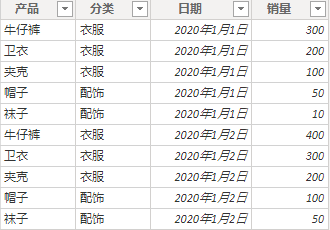（1）使用EVALUATE输出二维表的内容

EVALUATE
'销售表'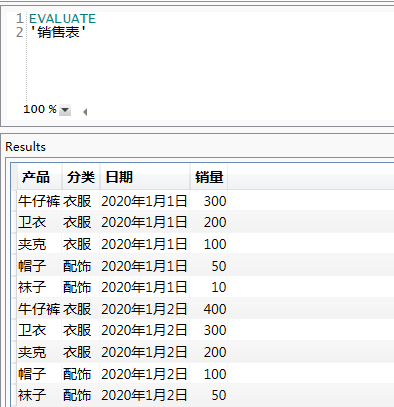（2）使用EVALUATE输出单个值的方法：将单个值转换为单行表

EVALUATE
row("列名",max('销售表'[销量]))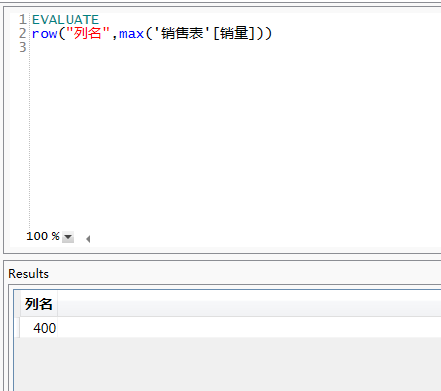DAX的编写有三种主要场景：新建度量值、新建列、新建表。下面分别叙述下涉及上下文的调试方法。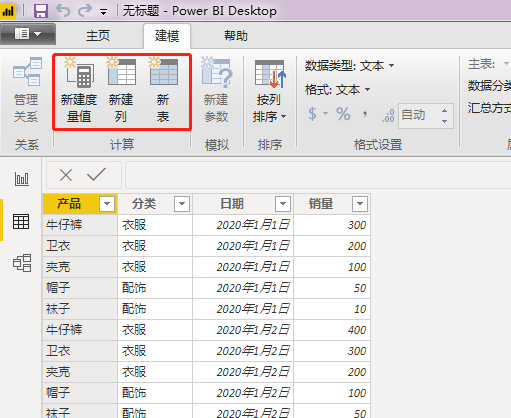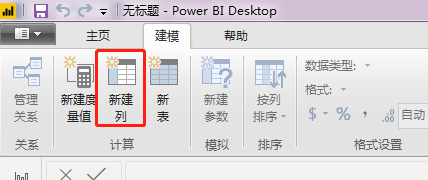EVALUATE
'表名',
"新增列名",新增列的dax公式
)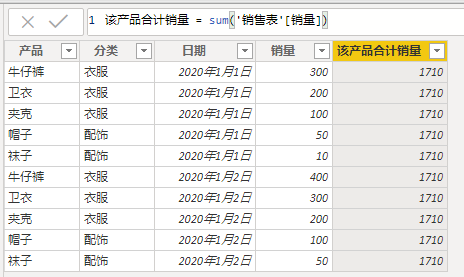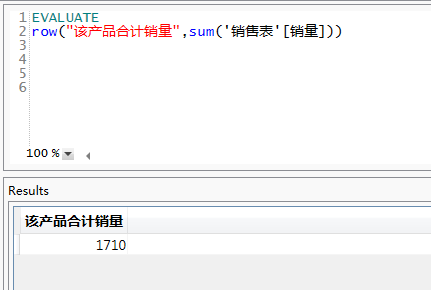EVALUATE
'销售表',
"该产品合计销量1", sum('销售表'[销量])
)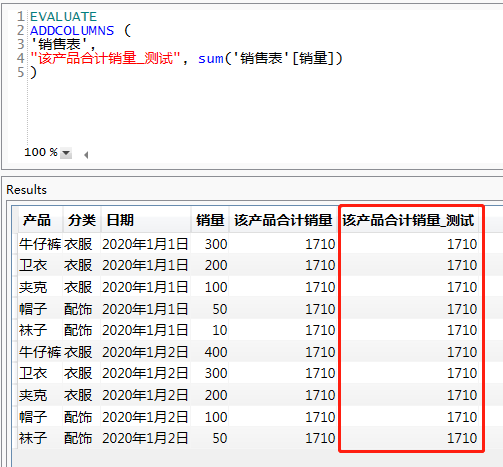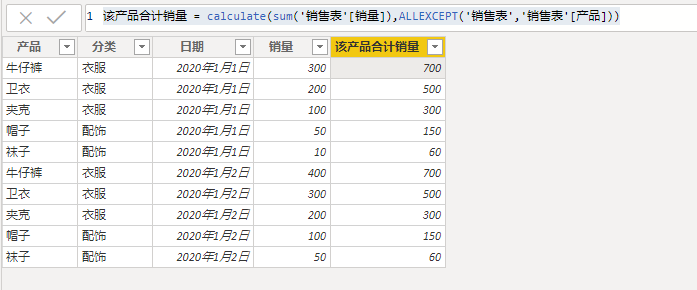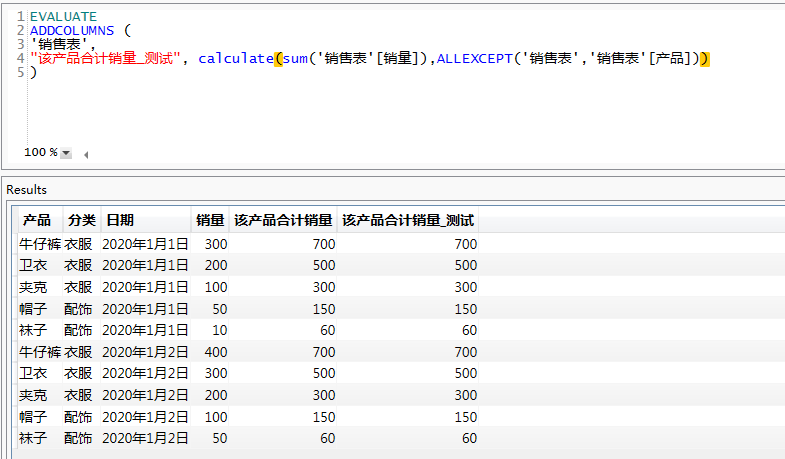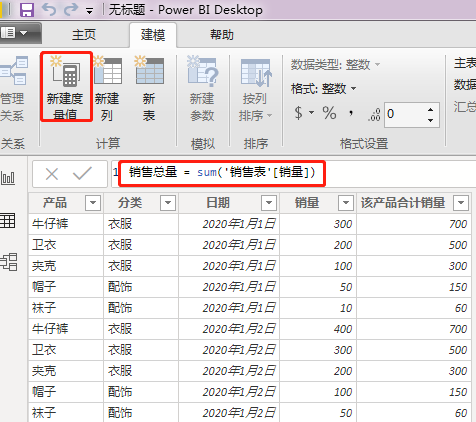（1）一维场景下使用度量值的测试

EVALUATE
SUMMARIZE('表名', '表名'[汇总字段],"度量值名称",度量值的dax公式)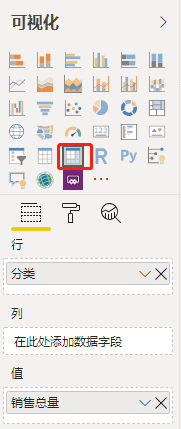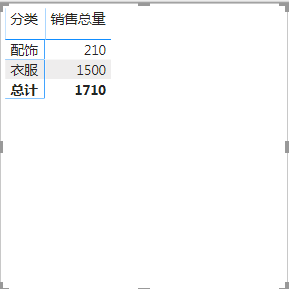EVALUATE
SUMMARIZE('销售表','销售表'[分类],"销售总量",sum('销售表'[销量]))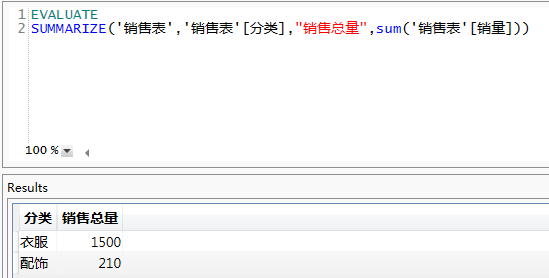（2）二维场景下使用度量值的测试

EVALUATE
SUMMARIZE(FILTER('表名', '表名'[列汇总字段]="字段值1"),[行汇总字段]," 列汇总字段",度量值的dax公式)

EVALUATE
SUMMARIZE(FILTER('表名', '表名'[列汇总字段]="字段值2"),[行汇总字段]," 列汇总字段",度量值的dax公式)

……

EVALUATE
SUMMARIZE(FILTER('表名', '表名'[列汇总字段]="字段值n"),[行汇总字段]," 列汇总字段",度量值的dax公式)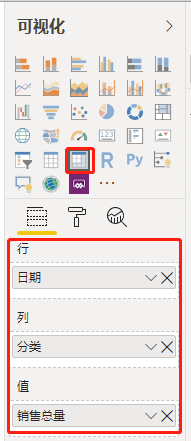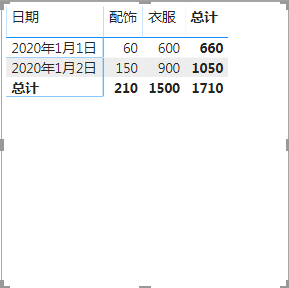EVALUATE
SUMMARIZE(FILTER('销售表','销售表'[分类]="配饰"),[日期],"配饰",sum('销售表'[销量]))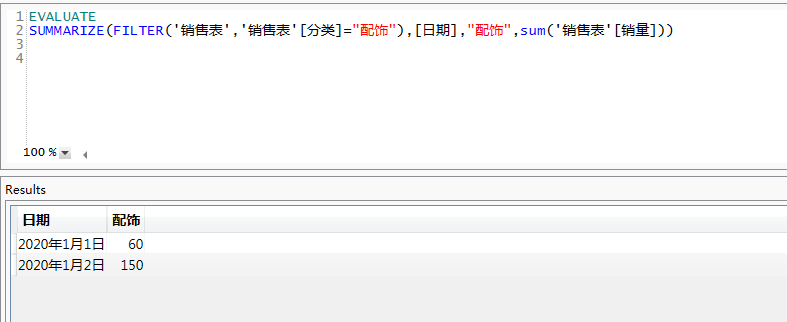EVALUATE
SUMMARIZE(FILTER('销售表','销售表'[分类]="配饰"),[日期],"配饰",sum('销售表'[销量]))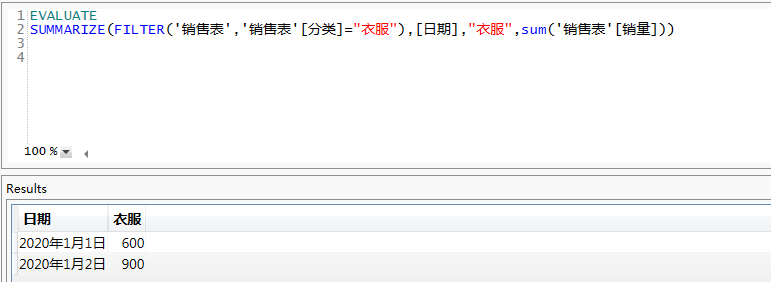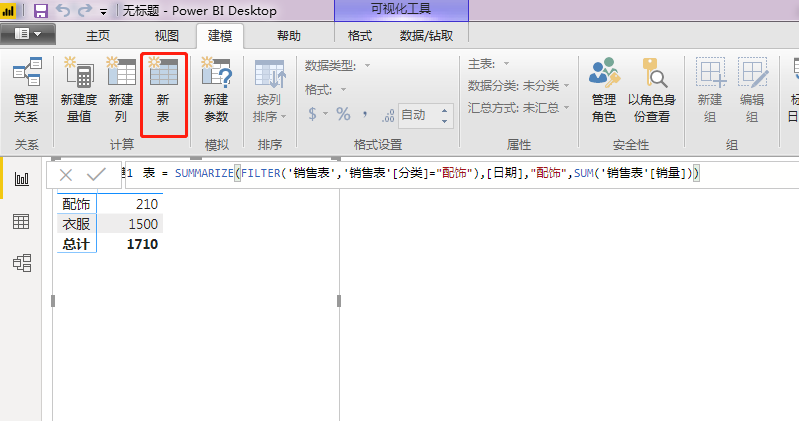©️2019 CSDN 皮肤主题: Age of Ai 设计师: meimeiellie